Next: Example 13.3: Converging lenses Up: Paraxial Optics Previous: Example 13.1: Concave mirrors

## Example 13.2: Convex mirrors

Question: How far must an object be placed in front of a convex mirror of radius of curvature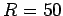cm in order to ensure that the size of the image is ten times less than the size of the object? How far behind the mirror is the image located?

Answer: The focal length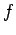of a convex mirror is minus half of its radius of curvature (taking the sign convention for the focal lengths of convex mirrors into account). Thus,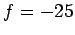cm. If the image is ten times smaller than the object then the magnification is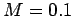. We can be sure that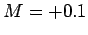, as opposed to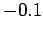, because we know that images formed in convex mirrors are always virtual and upright. According to Eq. (352), the image distanceis given by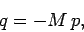where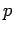is the object distance. This can be combined with Eq. (358) to give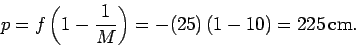Thus, the object must be placed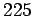cm in front of the mirror. The image distance is given by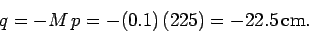Thus, the image is located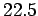cm behind the mirror.Next: Example 13.3: Converging lenses Up: Paraxial Optics Previous: Example 13.1: Concave mirrors
Richard Fitzpatrick 2007-07-14